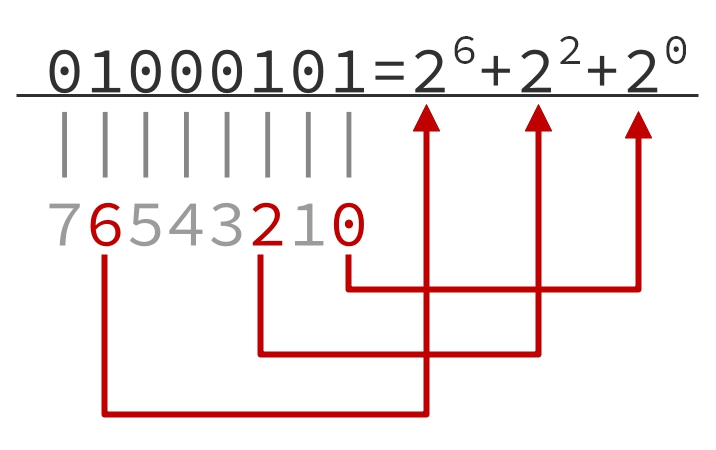# 树的最近公共祖先算法

## 性质¶

1. $$\text{LCA}(\{u\})=u$$
2. $$u$$$$v$$ 的祖先，当且仅当 $$\text{LCA}(u,v)=u$$
3. 如果 $$u$$ 不为 $$v$$ 的祖先并且 $$v$$ 不为 $$u$$ 的祖先，那么 $$u,v$$ 分别处于 $$\text{LCA}(u,v)$$ 的两棵不同子树中；
4. 前序遍历中，$$\text{LCA}(S)$$ 出现在所有 $$S$$ 中元素之前，后序遍历中 $$\text{LCA}(S)$$ 则出现在所有 $$S$$ 中元素之后；
5. 两点集并的最近公共祖先为两点集分别的最近公共祖先的最近公共祖先，即 $$\text{LCA}(A\cup B)=\text{LCA}(\text{LCA}(A), \text{LCA}(B))$$
6. 两点的最近公共祖先必定处在树上两点间的最短路上；
7. $$d(u,v)=h(u)+h(v)-2h(\text{LCA}(u,v))$$，其中 $$d$$ 是树上两点间的距离，$$h$$ 代表某点到树根的距离。

## 求法¶

### 倍增算法¶

#### 过程¶#### 性质¶

https://oi-wiki.org/graph/lca/ https://shawnliang.wiki/post/lca-binary-lifting/